GFG App
Open AppBrowser
Continue

In this article, we will see how we can make a simple app with Pygame.

Pygame is a library for python that helps us to build multimedia applications (Apps) and games. This library works as a wrapper for Simple DirectMedia Layer (SDL).

### Approach

• Create the app class
• Set Different windows.

## What are Classes?

A class is a template-like entity for creating objects. These class objects consist of varied behavior associated with them. In Pygame, we have different types of classes available they are App class, Text Class, and Scene Class. These in general have their own behavior and properties and can be used for varied purposes.

### App class

App class is the basis for a game template or any application that we are building. This class can also be called the base or the formation of an application or game. It allows various functionality like resizable, fullscreen, and no frame mode in Pygame thus it becomes the necessary class while declaring or building any app or game via Pygame in python.

Steps needed to form a base App class:

1. Importing the Pygame module.
2. Now, we initialize the App class.
3. Declare the main class and run the app class.

## Python3

 `# importing pygame and we can call it by using py` `import` `pygame as py`   `# importing all the libraries of pygame.local` `from` `pygame.``locals` `import` `*`   `# this is a simple App class in pygame`     `class` `App_Class:`   `    ``def` `__init__(``self``):`   `        ``# initializing the function for app` `        ``# class to declare the changeable content` `        ``py.init()` `        ``App_Class.screen ``=` `py.display.set_mode(` `            ``# size of window will be 540x340` `            ``(``540``, ``340``))` `        ``App_Class.running ``=` `True`   `    ``def` `play(``self``):` `        ``# this will run until the window is visible` `        ``while` `App_Class.running:` `            ``for` `event ``in` `py.event.get():` `              `  `                ``# check whether the cancel` `                ``# button is pressed or not` `                ``if` `event.``type` `=``=` `QUIT:` `                    ``App_Class.running ``=` `False`   `        ``py.quit()`   `if` `__name__ ``=``=` `'__main__'``:` `    ``App_Class().play()`

Output: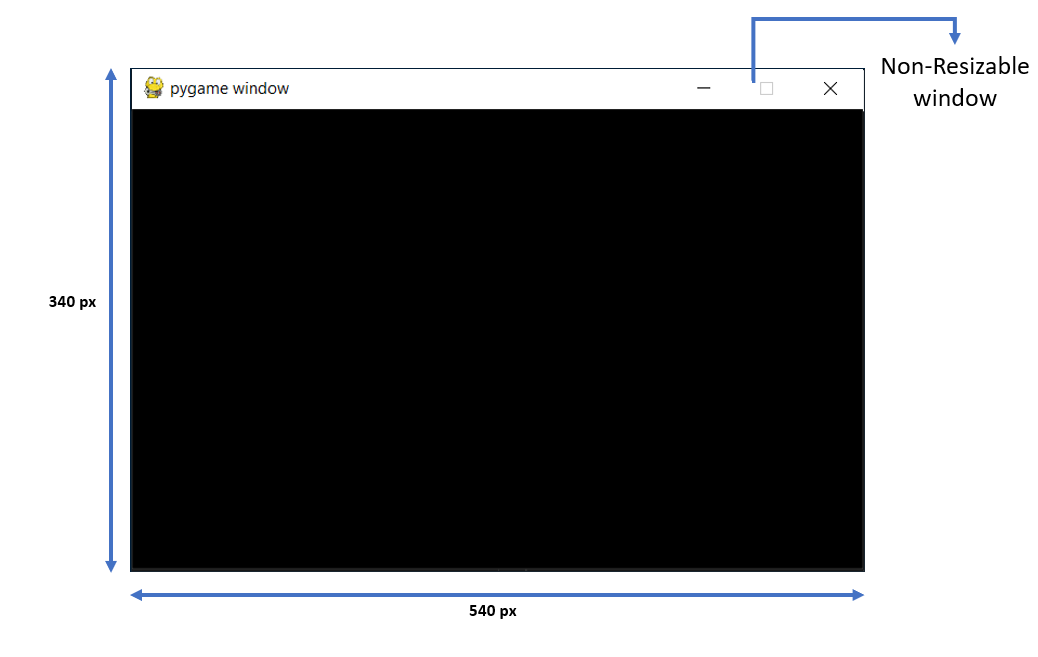App Class in pygame

## Text class

While building our application sometimes we want to display some messages for that, we required Text class. It is the template in which we can build different types of text formats in our window. This class mainly instantiates text objects of our window. So we can say if we want to play with our text then go for Text class.

Steps needed to form a Text class:

1. Import the Pygame module and all the libraries from the Pygame.
2. Now declare the text class and its functions like font_set, rendering, and previously used app class (As app class is the base class for all the other multimedia classes).
3. Finally, declare the main class for calling the run function of the app class that will run the test class feature in it.

## Python3

 `import` `pygame as py  ` `from` `pygame.``locals` `import` `*`    `class` `Text:`   `    ``def` `__init__(``self``, text, pos, ``*``*``options):` `        ``self``.text ``=` `text` `        ``self``.fontname ``=` `None` `        ``self``.pos ``=` `pos` `        ``self``.fontcolor ``=` `Color(``'Green'``)` `        `  `        ``# here 50 is the font size` `        ``self``.font ``=` `py.font.Font(``self``.fontname, ``50``)  `   `        ``# rendering the text in the window` `        ``self``.placing ``=` `self``.font.render(``self``.text, ``True``, ` `                                        ``self``.fontcolor)`   `        ``self``.rect ``=` `self``.placing.get_rect()`   `        ``# this fix the position of the text` `        ``self``.rect.bottomleft ``=` `self``.pos  `   `    ``def` `building(``self``):`   `        ``# put the text on the window` `        ``app.screen.blit(``self``.placing, ``self``.rect)`     `class` `app:`   `    ``def` `__init__(``self``):`   `        ``# Initialize all the parameters to build` `        ``# its base a blank window will appear` `        ``py.init()` `        ``app.screen ``=` `py.display.set_mode((``540``, ``340``))` `        ``app.t ``=` `Text(``'Text Class in pygame'``, pos``=``(``100``, ``150``))` `        ``app.running ``=` `True`   `    ``def` `run(``self``):`   `        ``# run function` `        ``while` `app.running:` `            ``for` `event ``in` `py.event.get():` `                ``if` `event.``type` `=``=` `QUIT:` `                    ``app.running ``=` `False`   `            ``app.screen.fill(Color(``'blue'``))`   `            ``app.t.building()`   `            ``py.display.update()`   `        ``py.quit()`   `# program runs from here` `if` `__name__ ``=``=` `'__main__'``:`   `    ``# calling function from the base class (app)` `    ``app().run()`

Output: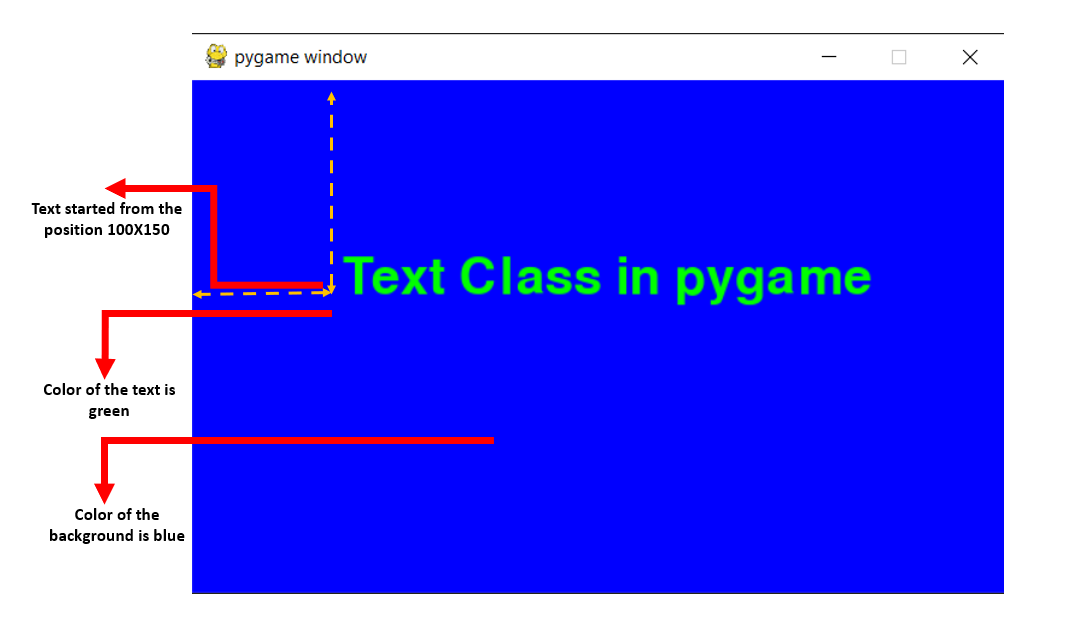### Scene Class

In games we have different screens, they might be the Intro scene, Game over the scene, or Level scene. In all these, we see different backgrounds or scenes. This all can be done with the help of the Scene class.

Steps needed to form a Scene class:

1. The first step is to declare the scene class. By doing this, we actually create a container in which we can store all the necessary features required for our game.
2. Now we have to append multiple scenes to the applications scene list a container.
3. After appending, we will add the scene id and the color for the multiple scenes. We will add a scene id so that we can easily call each scene according to the scene id.
4. Now it will flip each node and finally display the screen.
5. Representation of the scene is followed by their unique id given above.

## Python3

 `import` `pygame as py`   `title_py ``=` `"Scene 1 Starting scene"` `frame ``=` `50`   `# color setting for background and foreground` `green ``=` `(``0``, ``200``, ``0``)` `white ``=` `(``255``, ``255``, ``255``)`   `# this will set the font of the screen` `font_size ``=` `None`   `# building the windows and initialising it` `py.init()` `clock ``=` `py.time.Clock()` `screen ``=` `py.display.set_mode([``740``, ``340``])` `py.display.set_caption(title_py)`   `# calling and building the fonts` `screen_font ``=` `py.font.Font(font_size, ``50``)`   `# scene class in pygame` `class` `Scene():` `    ``def` `__init__(``self``):` `        ``self``.next_scene ``=` `self`   `    ``def` `process_input(``self``, events, press):` `        ``raise` `NotImplementedError`   `    ``def` `update(``self``):` `        ``raise` `NotImplementedError`   `    ``def` `rendering(``self``):` `        ``raise` `NotImplementedError`   `    ``def` `terminate(``self``):` `        ``self``.next_scene ``=` `None`   `# this will be the first scene class as` `# the prime and opening scene of our app` `class` `starting_scene(Scene):` `    ``def` `__init__(``self``):` `        ``super``().__init__()`   `    ``def` `process_input(``self``, events, press):` `        ``for` `event ``in` `events:` `            ``if` `event.``type` `=``=` `py.KEYDOWN:` `                ``if` `event.key ``=``=` `py.K_SPACE:` `                    ``self``.next_scene ``=` `EndScene()`   `    ``def` `rendering(``self``):` `        ``screen.fill(green)` `        ``text ``=` `screen_font.render(title_py, ``1``, white)` `        ``rect ``=` `text.get_rect()` `        ``rect.centerx ``=` `740` `/``/` `2` `        ``rect.centery ``=` `50` `        ``screen.blit(text, rect)`   `    ``def` `update(``self``):` `        ``pass`   `class` `EndScene(Scene):` `    ``def` `__init__(``self``):` `        ``super``().__init__()`   `    ``def` `process_input(``self``, events, press):` `        ``for` `event ``in` `events:` `            ``if` `event.``type` `=``=` `py.KEYDOWN:` `                ``if` `event.key ``=``=` `py.K_SPACE:` `                    ``self``.next_scene ``=` `starting_scene()`   `    ``def` `update(``self``):` `        ``pass` `    ``# rendering the scene function` `    ``def` `rendering(``self``):` `        ``screen.fill(green)` `        `  `        ``# font color will be white` `        ``text ``=` `screen_font.render(``"Scene 2 Game Ending "``, ``1``, white)` `        ``rect ``=` `text.get_rect()` `        ``rect.centerx ``=` `370`  `# location from x-axis` `        ``rect.centery ``=` `50`  `# location from y-axis` `        ``screen.blit(text, rect)`   `class` `app_class():` `    ``def` `__init__(``self``):` `        ``self``.running_scene ``=` `starting_scene()`   `    ``def` `control(``self``, event, press):` `        ``x_out ``=` `event.``type` `=``=` `py.QUIT` `        ``quit ``=` `press[py.K_q]` `        `  `        ``# if anyone click on the cross` `        ``# button or press the 'q' button ` `        ``# it will quit the window` `        ``return` `x_out ``or` `(quit)`   `    ``def` `run(``self``):` `        ``while` `self``.running_scene !``=` `None``:` `            ``eve ``=` `[]` `            ``press ``=` `py.key.get_pressed()` `            ``for` `event ``in` `py.event.get():` `                ``if` `self``.control(event, press):` `                    ``self``.running_scene.terminate()` `                ``else``:` `                    ``eve.append(event)`   `            ``# Manage scene` `            ``self``.running_scene.process_input(eve, press)` `            ``self``.running_scene.update()` `            `  `            ``# dont move it as first we need to update then render` `            ``self``.running_scene.rendering()` `            `  `            ``# moving the scene one by one` `            ``self``.running_scene ``=` `self``.running_scene.next_scene  ` `            `  `            ``# means it will allow user to change the scene` `            ``py.display.flip() ` `            ``# Update and tick` `            ``clock.tick(frame)`   `# main (our code will run from here)` `if` `__name__ ``=``=` `"__main__"``:` `    ``let_check ``=` `app_class()` `    ``let_check.run()` `    ``py.quit()`

Output:

Press the space bar to change the scene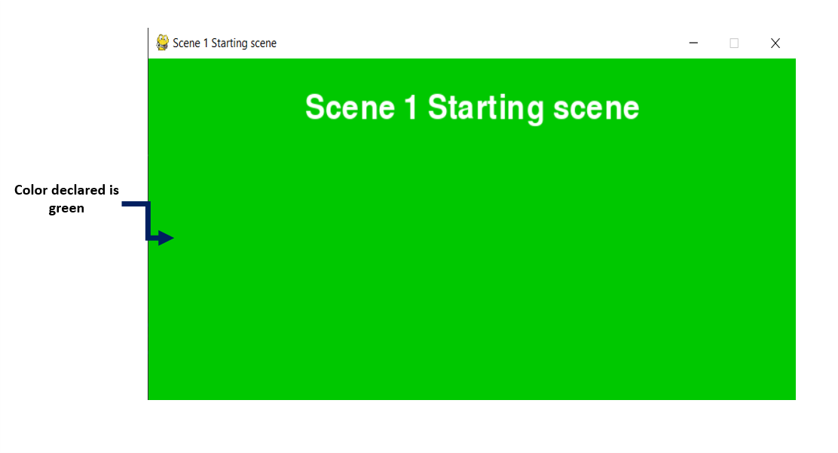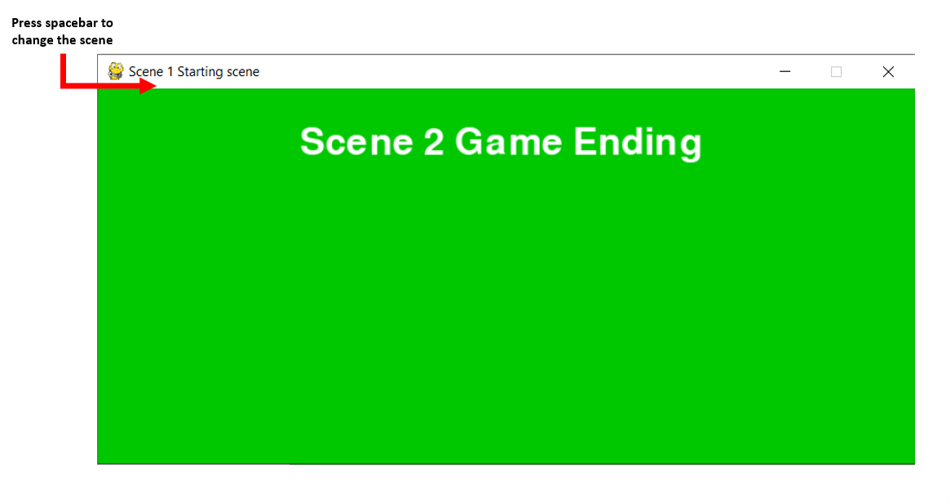scene2

## Different Windows modes

There are three different modes of a window in Pygame in which an active window can be displayed they are:

1. Fullscreen mode(As its name suggests it is a window with its full resizable capability)
2. Resizable mode(resize is allowed in this type of mode)
3. No frame mode(In this type of mode no title bar is present in the window)

Steps needed to set up these modes:

• First, we declare screen size and display mode flags inside the initialized app class method.
• Now we will create a screen surface for it
`pygame_screen.init()`
• Creating surface object
```# for FULLSCREEN mode
display_surface = pygame_screen.display.set_mode((500,250), FULLSCREEN)```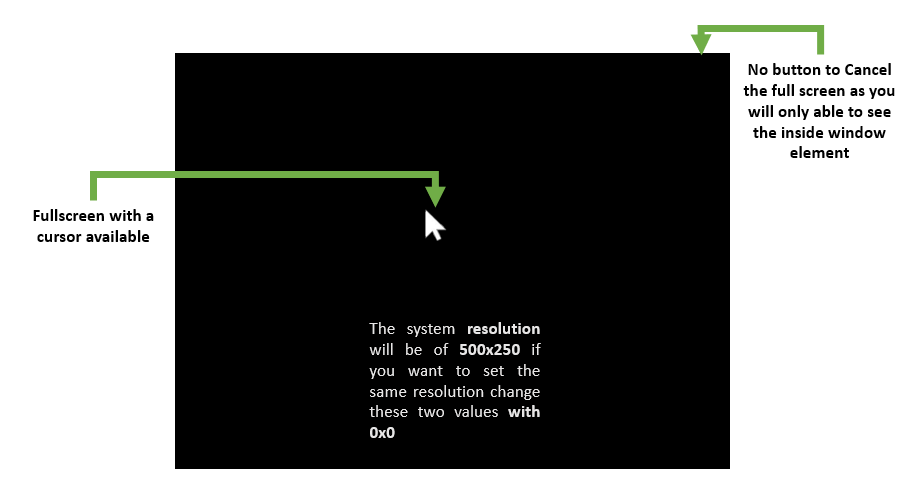FULLSCREEN mode in pygame

```# for RESIZABLE mode
display_surface = pygame_screen.set_mode((500, 250), RESIZABLE)```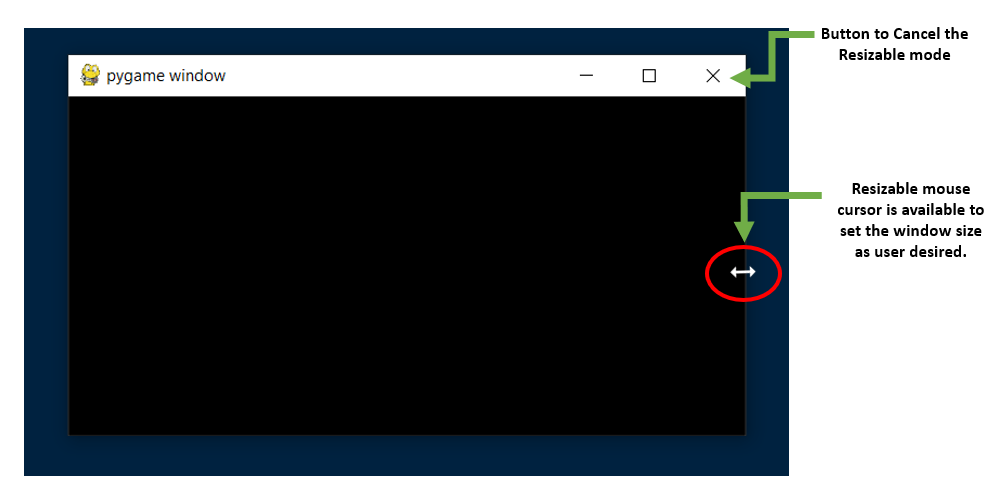The RESIZABLE mode in pygame

```# for NOFRAME mode
display_surface = pygame_screen.display.set_mode((500, 250), NOFRAME)```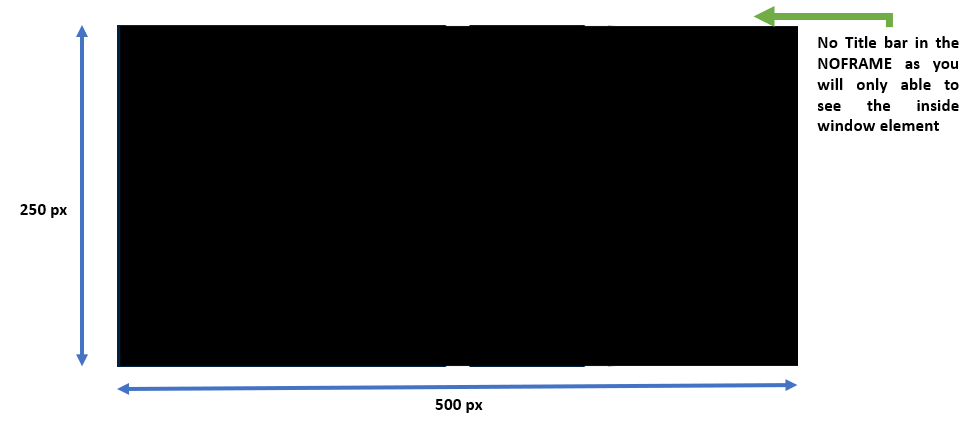NOFRAME mode in pygame

• Now run the main loop to execute the QUIT and Update command for the screen

## Python3

 `import` `pygame as py` `from` `pygame.``locals` `import` `*`   `# Create a displace surface object` `py.init()`   `# Un-comment one of the following` `# three line of code for varied mode of pygame` `# display_surface = py.display.set_mode((500, 250), NOFRAME)` `# display_surface = py.display.set_mode((500, 250), FULLSCREEN)` `display_surface ``=` `py.display.set_mode((``500``, ``250``), RESIZABLE)`   `working ``=` `True` `while` `working:  ``# run until it become false` `    ``for` `event ``in` `py.event.get():` `        ``if` `event.``type` `=``=` `py.QUIT:` `            ``working ``=` `False` `    ``py.display.update()`   `py.quit()  ``# quit the window`

Output: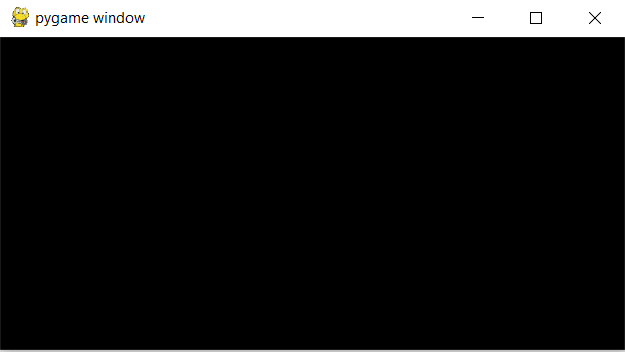### Node Placement:

Node act as a container for graphical user Interface elements and objects.  Node placement in Pygame requires four parameters that are:

• size: this helps to find the current size of the node on the screen
• pos: this helps to find the current position of the node
• dir: dir is a short name for direction. It itself consists of 3 parameters that are vertical, horizontal, and diagonal.
• gap: This refers to the space given in between two nodes in a Pygame.

My Personal Notes arrow_drop_up